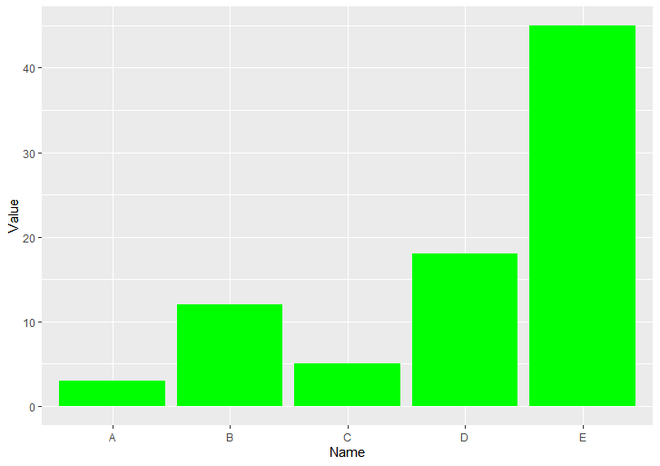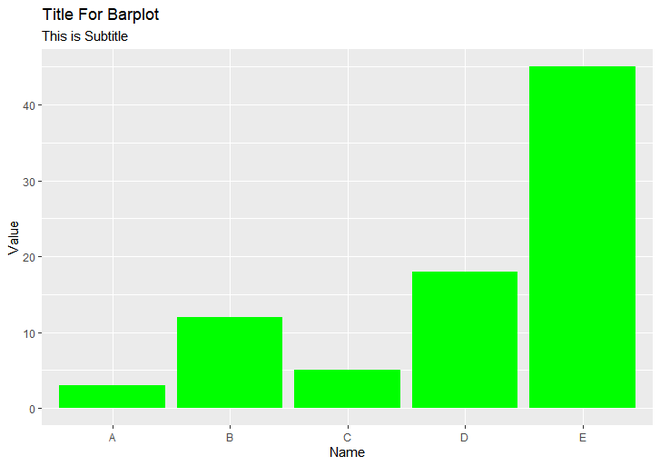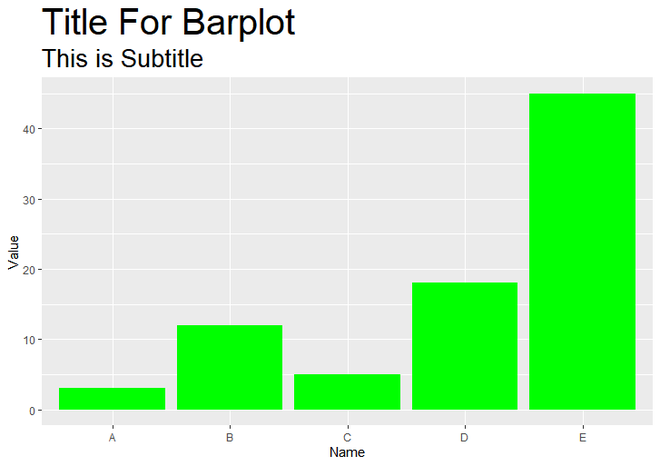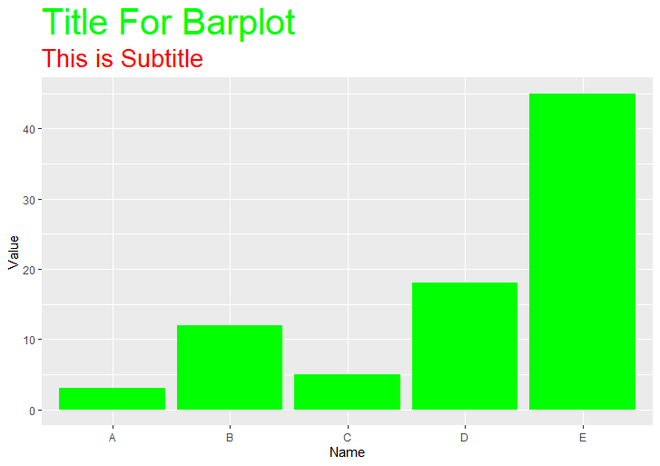# ggplot2 – Title and Subtitle with Different Size and Color in R

• Last Updated : 16 May, 2021

A Title and the subtitle to a plot give a piece of information about the graph that what the graph actually wants to represent. This article describes how to add a Title and Subtitle with Different Sizes and Colors using ggplot2 in R Programming.

To add a Title and Subtitle within a plot, first, we have to import ggplot2 library using library() function. If you have not installed yet, you can simply install it by writing a command install.packages(“ggplot2”) in R Console.

`library(ggplot2)`

Consider the following data for the example:

```data <- data.frame(
name=c("A","B","C","D","E") ,
value=c(3,12,5,18,45)
)```

Creating a Plot using ggplot() function with the value of X-axis as Name and Y-axis as Value and make it a barplot using geom_bar() function of the ggplot2 library. Here we use the fill parameter to geom_bar() function to color the bars of the plots.

## R

 `# Load Package ` `library``(ggplot2) ` ` `  `# Create a Data ` `data <- ``data.frame``( ` `  ``Name=``c``(``"A"``, ``"B"``, ``"C"``, ``"D"``, ``"E"``) ,   ` `  ``Value=``c``(3, 12, 5, 18, 45) ` `) ` ` `  `# Create a Simple BarPlot with green color. ` `ggplot``(data, ``aes``(x = Name, y = Value)) +  ` `  ``geom_bar``(stat = ``"identity"``, fill = ``"green"``) `

Output:## Adding Title and Subtitle To R Plot

Method 1. By Using ggtitle() function:

For this, we simply add ggtitle() function to a geom_bar() function. Inside ggtitle() function, we can directly write the title that we want to add to the plot without defining any parameter but for add Subtitle to the plot using ggtitle() function, we have to use subtitle parameter to ggtitle() function and then assign the subtitle to that parameter.

Syntax : ggtitle(“Title of the Plot”, subtitle = “Subtitle of the Plot”)

Parameter :

• we give title that we want to add, as it’s parameter.
• subtitle is used as a second parameter of ggtitle() function to add subtitle of plot.

Below is the implementation:

## R

 `# Load Package ` `library``(ggplot2) ` ` `  `# Create a Data ` `data <- ``data.frame``( ` `  ``Name=``c``(``"A"``, ``"B"``, ``"C"``, ``"D"``, ``"E"``), ` `  ``Value=``c``(3, 12, 5, 18, 45) ` `) ` ` `  `# Create a BarPlot and add title ` `# and subtitle to it using ggtitle() function. ` `ggplot``(data, ``aes``(x = Name, y = Value)) +  ` `  ``geom_bar``(stat = ``"identity"``, fill = ``"green"``)+ ` `  ``ggtitle``(``"Title For Barplot"``, ` `       ``subtitle = ``"This is Subtitle"` `       ``) `

Output:Method 2. By Using labs() Function:

To add Title and Subtitle to R Plot using labs() function, things are same as above only difference is we use labs() function instead of ggtitle() function and assign title that we want to add to the parameter called ‘title’. Subtitle can be added using the same parameter of the above example. Output is also the same as the above example output.

Syntax : ggtitle(“Title of the Plot”, subtitle = “Subtitle of the Plot”)

Parameter :

• title is used as a first parameter to add the title of Plot.
• subtitle is used as a second parameter to add the subtitle of Plot.

Below is the implementation:

## R

 `# Load Package ` `library``(ggplot2) ` ` `  `# Create Data ` `data <- ``data.frame``( ` `  ``Name = ``c``(``"A"``, ``"B"``, ``"C"``, ``"D"``, ``"E"``) ,   ` `  ``Value = ``c``(3, 12, 5, 18, 45) ` `) ` ` `  `# Create BarPlot and add title ` `# and subtitle to it using labs() function. ` `ggplot``(data, ``aes``(x = Name, y = Value)) +  ` `  ``geom_bar``(stat = ``"identity"``, fill = ``"green"``)+ ` `  ``labs``(title = ``"Title For Barplot"``, ` `       ``subtitle = ``"This is Subtitle"` `       ``) `

Output:## Title and Subtitle With Different Size

To change the size of the title and subtitle, we add the theme() function to labs() or ggtitle() function, whatever you used. Here we use labs() function. Inside theme() function, we use plot.title parameter for doing changes in the title of plot and plot.subtitle for doing changes in Subtitle of Plot. We use element_text() function as a value of plot.title and plot.subtitle parameter. We can change the appearance of texts using element_text() function. To change the size of the title and subtitle, we use the size parameter of element_text() function. Here we set the size of the title as 30 and the size of the subtitle as 20.

Below is the implementation:

## R

 `library``(ggplot2) ` ` `  `data <- ``data.frame``( ` `  ``Name = ``c``(``"A"``, ``"B"``, ``"C"``, ``"D"``, ``"E"``) ,   ` `  ``Value=``c``(3, 12, 5, 18, 45) ` `) ` ` `  `# Create a BarPlot with title ` `# of size 30 and subtitle of size 20 ` `ggplot``(data, ``aes``(x = Name, y = Value)) +  ` `  ``geom_bar``(stat = ``"identity"``, fill = ``"green"``)+ ` `  ``labs``(title = ``"Title For Barplot"``, ` `       ``subtitle = ``"This is Subtitle"` `       ``)+ ` `  ``theme``(plot.title = ``element_text``(size = 30), ` `        ``plot.subtitle = ``element_text``(size = 20) ` `        ``) `

Output:Title and Subtitle with Different size

## Title and Subtitle With Different Color

To change the color of Title and Subtitle, We simply add a color parameter to element_text() function. All others are the same as the above implementation. Here we set the value of the color of title as green and the value of subtitle as red.

## R

 `library``(ggplot2) ` ` `  `data <- ``data.frame``( ` `  ``Name = ``c``(``"A"``, ``"B"``, ``"C"``, ``"D"``, ``"E"``) ,   ` `  ``Value = ``c``(3, 12, 5, 18, 45) ` `) ` ` `  `# Create a BarPlot with title ` `# and subtitle with different colors. ` `ggplot``(data, ``aes``(x = Name, y = Value)) +  ` `  ``geom_bar``(stat = ``"identity"``, fill = ``"green"``)+ ` `  ``labs``(title = ``"Title For Barplot"``, ` `       ``subtitle = ``"This is Subtitle"` `       ``)+ ` `  ``theme``(plot.title = ``element_text``(size = 30, color = ``"green"``), ` `        ``plot.subtitle = ``element_text``(size = 20, color = ``"red"``) ` `        ``) `

Output:Title and Subtitle with Different Color

My Personal Notes arrow_drop_up
Recommended Articles
Page :